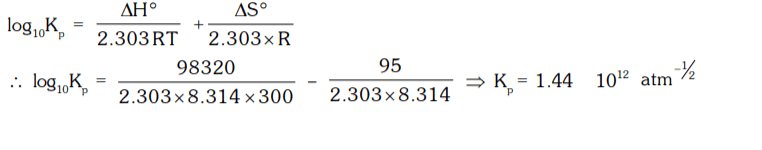## Chemical Equilibrium Questions and Answers Part-2

1. A mixture of H2 and I2 in molecular proportion of 2 : 3 was heated at 444°C till the reaction H2 + I2 ⇌ 2HI reached equilibrium state. Calculate the percentage of iodine converted into HI. (KC at 444°C is 0.02)
a) 4.38%
b) 6.38%
c) 5.38%
d) 3.38%

Explanation:2. 2SO2(g) + O2(g) ⇌ 2SO3 (g)
If the partial pressure of SO2, O2 and SO3 are 0.559, 0.101 and 0.331 atm respectively. What would be the partial pressure of O2 gas, to get equal moles of SO2 and SO3.
a) 0.288 atm
b) 0.388 atm
c) 0.488 atm
d) 0.188 atm

Explanation:3. The equilibrium constant of the reaction A2 (g) + B2(g) ⇌ 2AB (g) at 100°C is 50. If one litre flask containing one mole of A2 is connected to a 3 litre flask containing two moles of B2 the number of moles of AB formed at 373 K will be -
a) 0.943
b) 18.86
c) 2.317
d) 1.886

Explanation:4. The equilibrium constant K, for the reaction N2 + 3H2 ⇌ 2NH3 is 1.64 × 10-4 atm-2 at 300°C. What will be the equilibrium constant at 400°C, if heat of reaction in this temperature range is – 105185.8 Joules.
a) 0.64 × 10-5 atm-2
b) 6.4 × 10-3 atm-2
c) 0.64 × 10-3 atm-2
d) 0.64 × 10-1 atm-2

Explanation:5. When 1.0 mole of N2 and 3.0 moles of H2 was heated in a vessel at 873 K and a pressure of 3.55 atm. 30% of N2 is converted into NH3 at equilibrium. Find the value of KP for the reaction.
a) 4.1 × 10-2 atm-2
b) 5.1 × 10-2 atm-2
c) 6.1 × 10-2 atm-2
d) 3.1 × 10-2 atm-2

Explanation:6. A2(g) and B2(g) at initial partial pressure of 98.4 and 41.3 torr, respectively were allowed to react at 400 K. At equilibrium the total pressure was 110.5 torr. Calculate the value of KP for the following reaction at 400 K.
2A2(g) + B2(g) ⇌ 2 A2B (g)
a) 134
b) 154
c) 124
d) 174

Explanation:7. Ammonium hydrogen sulphide dissociated according to the equation, NH4HS (s) ⇌ NH3(g) + H2S (g). If the observed pressure of the mixture is 2.24 atm at 106°C, what is the equilibrium constant Kp of the reaction ?
a) 3.2764 atm2
b) 1.2544 atm2
c) 2.3247 atm2
d) 8.1267 atm2

Explanation:8. For the reaction, CaCO3(s) ⇌ CaO(s) + CO2 (g) ; K = 0.059 atm at 1000 K. 1 g of CaCO3 is placed in a 10 litre container at 1000 K to reach the equilibrium. Calculate the mass of CaCO3 left at equilibrium.
a) 0.38 g
b) 0.18 g
c) 0.28 g
d) 0.72 g

Explanation:9. In a mixture of N2 and H2, initially they are in a mole ratio of 1 : 3 at 30 atm and 300°C, the percentage of ammonia by volume under the equilibrium is 17.8%. Calculate the equilibrium constant (Kp) of the mixture, for the reaction, N2(g) + 3H2(g) ⇌ 2NH3(g).
a) 3.61 × 10-4 atm-2
b) 5.47 × 10-4 atm-2
c) 2.24 × 10-4 atm-2
d) 7.31 × 10-4 atm-2

Explanation:10. Given below are the values of ∆H° and ∆S° for the reaction at 27°C,
SO2(g) + 1/2 O2(g) ⇌ SO3(g)
∆H° = 98.32 kJ/mol
∆S° = –95 J/mol. Calculate the value of Kp for the reaction.
a) 1.44 × 1012 atm-1/2
b) 2.39 × 1012 atm-1/2
c) 7.52 × 1012 atm-1/2
d) 4.61 × 1012 atm-1/2